Latest Banking jobs   »

# Quantitative Aptitude Quiz For IRDA AM 2023 -12th May

Directions (1-5): Given below are two equations which you have to solve for the value of x and y and give answer
(a) if x >y
(b) if x < y
(c) if x = y or no relation between x and y exist
(d) if x ≥ y
(e) if x ≤ y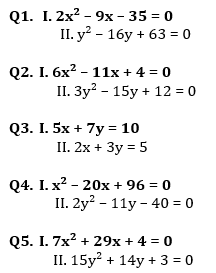Directions (6-10): In each of these questions, two equations (I) and (II) are given. You have to solve both the equations and give answer
(a) if x>y
(b) if x≥y
(c) if x<y
(d) if x ≤y
(e) if x = y or no relation can be established between x and y.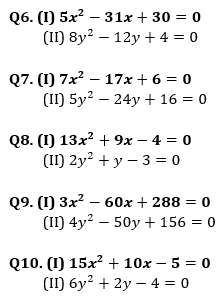Solutions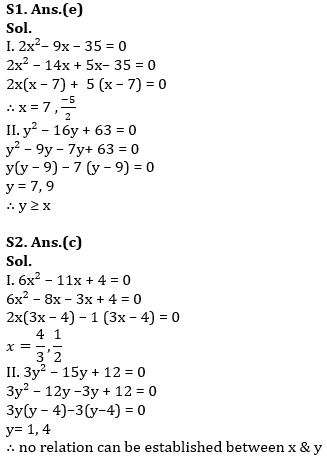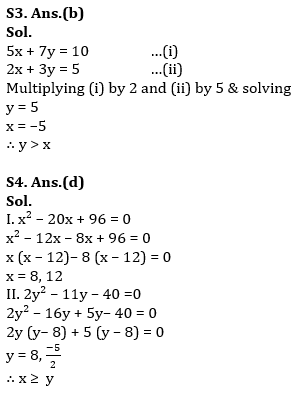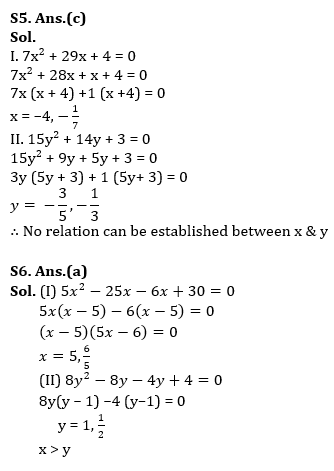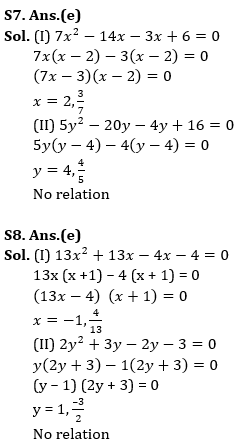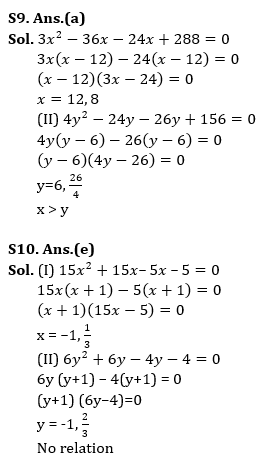.

## FAQs

### How many sections are there in the IRDAI exam?

There are 4 sections in the IRDAI assistant manager online preliminary exam. They are Reasoning, English Language, General awareness, Quantitative aptitude.

#### Congratulations!Union Budget 2023-24: Free PDF